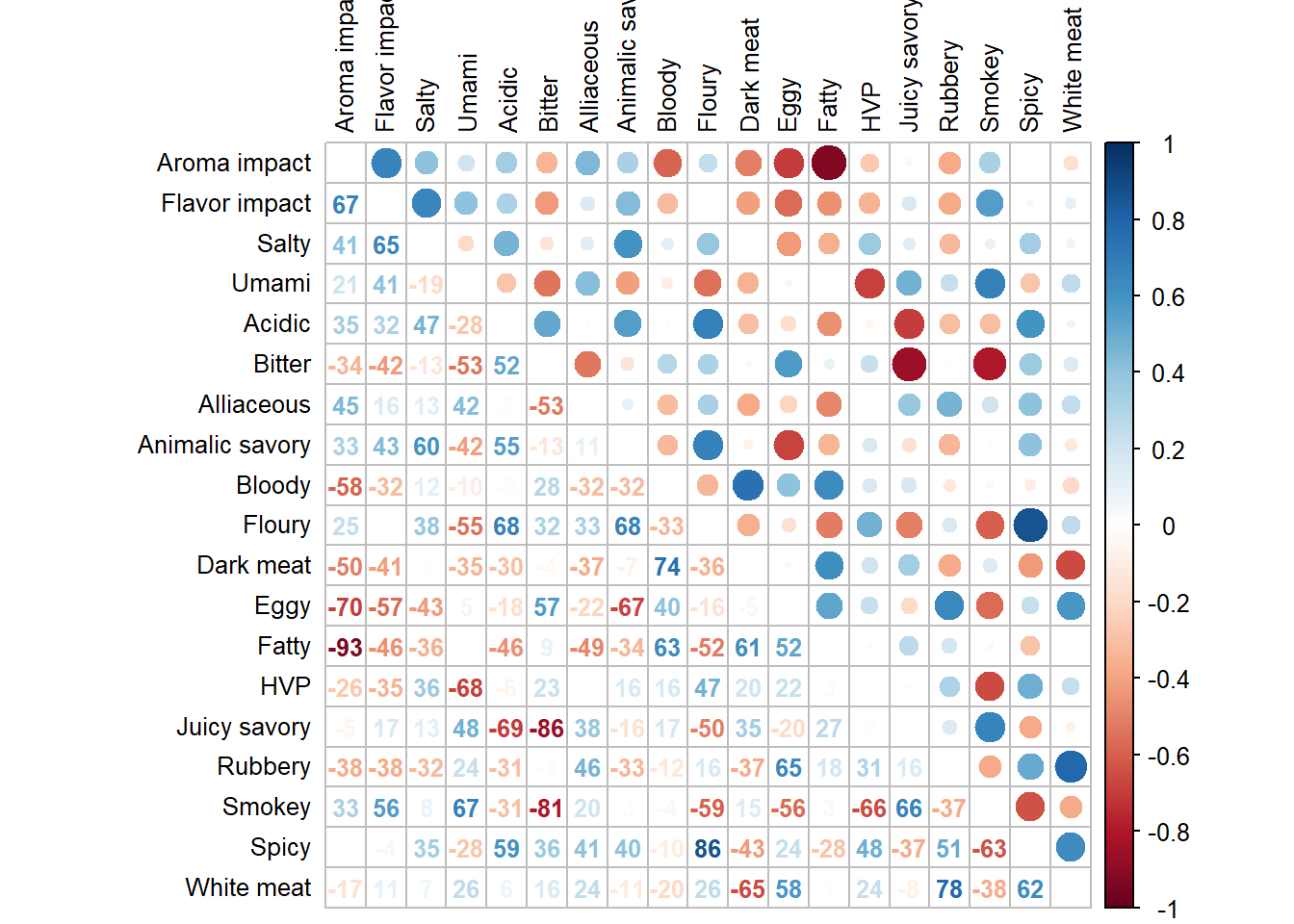## 3.2Heat Map

Comparing to the Correlation plot from PCA, we noticed that our Heat Map this time has a more pronounced correlation value. I speculate that this because using Group Means as data reduces the amount of variances. We see both positive and negative correlations among variables.

``````#heat map of means
#correlation plot 3: mixed circle visualization (top) and numbers (bottom)

corrplot3 <- corrplot.mixed(cor(sausage.processed.mean.prod), upper = 'circle',
lower = "number",
tl.pos = "lt",
tl.col = "black",
tl.cex = 0.8,
addCoefasPercent = TRUE,
number.cex=0.8)````````````#corrplot3
corrplot3 <- recordPlot()``````